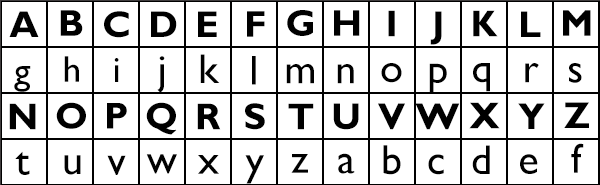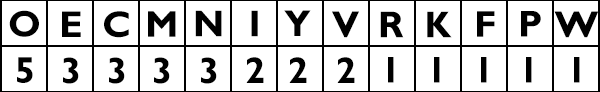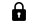# Caesar Cipher

The Caesar Cipher, also known as the Shift Cipher, is a very simple method of encoding a message.

The person encoding the cipher uses a shifted version of the alphabet to write out their message. This means that each letter of the alphabet is replaced with a different letter for the purposes of writing the cipher. For example, for a cipher with a shift of 5, the letter A would become F, B would become G and so on.

To encode the message I AM A CIPHER with a shift of 6, we would write out the alphabet twice, first normally and then beneath it shifting the alphabet 6 places to the left:The encoded message would therefore be:

O GS G IOVNKX

However, to decode a message that’s been encrypted using a shift cipher we have to work backwards.

Let’s imagine we have been given the code:

IYERKFOCEMMOCCPEVVINOMYNONWO

Now we know this is a Caesar cipher, so we can just start working through different shifts until we get the correct answer. But let’s imagine we didn’t know the type of cipher it was. What tactics can we quickly use to help us work out the cipher.

We ca look at the frequency of the letters in the message:From the Substitution Page we know that the most common letters are, in order, E, T, A, O, I, N.

Let's assume the most frequent letter in the cipher, O is therefore 'e', which would give us a shift of 10. That would mean that E='u', C='s', M='c' and N='d'.

None of these are amongst the most common letters, which means on first glance this would seem like an unlikely solution. However, there are three pairs of double letters in the code MM, CC and VV. The most common double letter in English is LL, followed by SS, EE, OO and TT. If O='e', then VV='ll', and we already know that with this solution CC='ss' and MM='cc', which is less common but not unheard of. Of course there is no guarantee that these double letters are in the same word - for example one word might end with 'c' and the next word begins with 'c'.

Having looked at those two factors let's assume that the shift of 10 is correct and apply it to the rest of the cipher.

This gives us the solution:

YOUHAVESUCCESSFULLYDECODEDME

Congratulations, you have learnt how to decode a Caesar cipher!